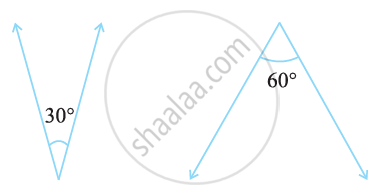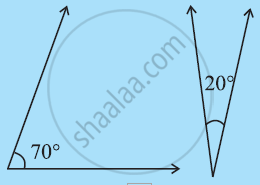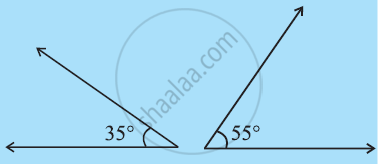# Related Angles - Complementary Angles

## Definition

Complementary angles: When the sum of the measures of two angles is 90°, the angles are called complementary angles. Example, 30° + 60° = 90°.

## Notes

### Complementary Angles:

• When the sum of the measures of two angles is 90°, the angles are called complementary angles.

• Example, 30° + 60° = 90°

• The ‘30° angle’ is the complement of the‘60° angle’ and vice versa.Some more Examples,## Example

What is the measure of the complement of the following angles?

45º

Let the other measure of the angle is xº.

The sum of the measures of complementary angles is 90º.

xº + 45º = 90º

xº = 90º - 45º

xº = 45º.

## Example

What is the measure of the complement of the following angles?

65º

Let the other measure of the angle is xº.

The sum of the measures of complementary angles is 90º.

xº + 65º = 90º

xº = 90º - 65º

xº = 25º.

## Example

The difference in the measures of two complementary angles is 12o. Find the measures of the angles.

∠1 - ∠2 = 12°

⇒ ∠1 - (90° - ∠1) = 12°

⇒ ∠1 - 90° + ∠1 = 12°

⇒ 2∠1 - 90° = 12°

⇒ 2∠1 = 12° + 90°

⇒ 2∠1 = 102°

⇒ ∠1 = (102°)/2

⇒ ∠1 = 51°

∠1 + ∠2 = 90°
⇒ ∠2 = 90° - ∠1

⇒ ∠2 = 90° - 51°

⇒ ∠2 = 39°.

## Example

Find the measure of the complement of an angle of measure 70°?

Let the measure of the complementary angle be x.
70 + x = 90
∴ 70 + x - 70 = 90 - 70
∴ x = 20°
The measure of the complement of an angle of measure 70° is 20°

## Example

Angles of measures (a + 15)° and (2a)° are complementary. What is the measure of each angle?

a + 15 + 2a = 90
3a + 15 = 90
3a = 75
a = 25
∴ a + 15 = 25 + 15 = 40°
and 2a = 2 × 25 = 50°.

If you would like to contribute notes or other learning material, please submit them using the button below.

### Shaalaa.com

What are Complementary Angles? [00:08:10]
S
0%